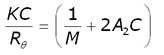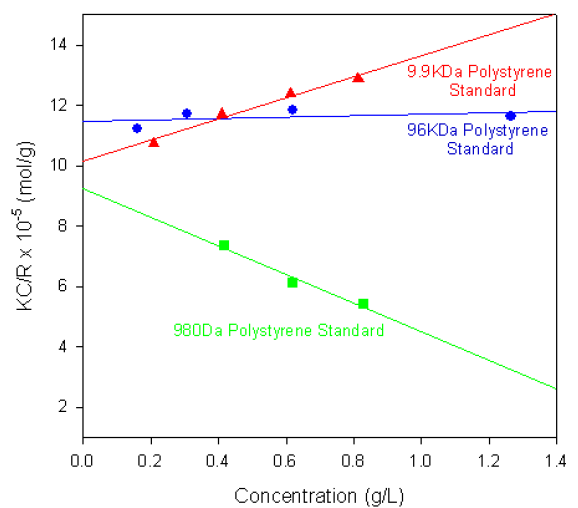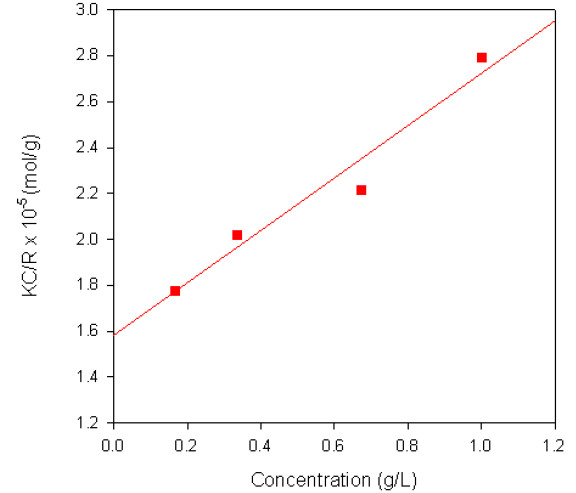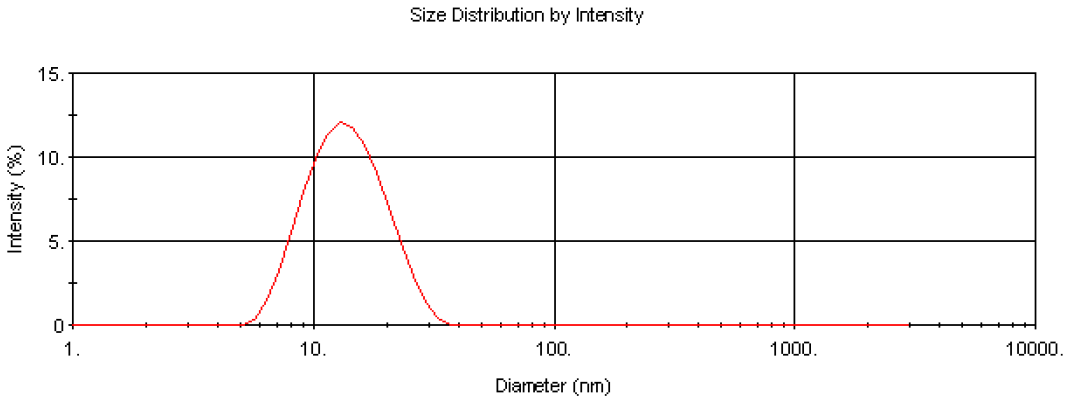# Polymers and Polysaccharides Including the Molecular Weight Determination of Polymers and Polysaccharides Using the Zetasizer Nano

The Zetasizer Nano System combines dynamic, static and electrophoretic light scattering technologies enabling for the measurement of particle size, zeta potential and molecular weight.

## Particle Size Determination by Static Light Scattering

Static light scattering (SLS) is a non-invasive technique used for characterizing macromolecules in solution. A beam of monochromatic light is directed through a sample and the intensity of the light scattered at an angle of 173° by the molecules is measured. SLS makes use of the time-averaged intensity of scattered light, from which the weight-averaged molecular weight and second virial coefficient can be determined.

The intensity of scattered light that a macromolecule produces is proportional to the product of the weight-average molecular weight and the concentration of the macromolecule. For molecules that show no angular dependence in their scattering, the relationship between the intensity of scattered light and their molecular weight is given by the Rayleigh equation:where K is an optical constant, Rθ is the Rayleigh ratio of the scattered to incident light intensity, M is the weight-average molecular weight, A2 is the second virial coefficient and C is the sample concentration.

Therefore, a plot of KC/Rθ versus C is expected to be linear with an intercept equivalent to 1/M and a slope equal to the second virial coefficient A2. Such a plot is known as a Debye plot.

The second virial coefficient is a property describing the interaction strength between the molecule and the solvent. For samples where A2 > 0, the molecules tend to stay in solution. When A2 = 0, the molecule-solvent interaction strength is equivalent to the molecule-molecule interaction strength and the solvent is described as being a theta solvent. When A2<0, the molecules will tend to crystallize or aggregate.

Further details of the theory of molecular weight determinations from static light scattering measurements can be found in other application notes available from the Malvern Panalytical website.

This application note details molecular weight measurements performed on various proteins, polymers and polysaccharides on the Zetasizer Nano.

## Requirements For Molecular Weight Measurements

• Zetasizer Nano S or ZS (instruments that incorporate NIBS™ optics)
• Quartz cuvette
• Preparation of a number of concentrations of the unknown molecule (protein or polymer) in a suitable solvent
• Determination of the differential refractive index increment (dñ/dC). This can either be found from literature sources or measured using a suitable refractometer. The dñ/dC value for globular proteins, for example, is 0.185 ml/g
• Measurement of the intensity of scattering from a standard sample whose Rayleigh Ratio is known. This is recommended to be toluene as the value is known at various wavelengths. For example, the Rayleigh ratio of toluene at 633 nm is 1.3522 x 10-5 cm-1
• Measurement of the intensity of scattering from the zero concentration sample (if the solvent is different to toluene)
• Measurement of the intensity of scattering from the various concentrations of sample

It should be noted that the Zetasizer Nano software measures the intensities of the scattered light of the prepared samples and automatically calculates the molecular weight and second virial coefficient from the data using a Debye plot.

## Polymers

Figure 1 shows the single-angle Debye plots for a series of polystyrene polymer standards prepared in toluene and measured on a Zetasizer Nano S. The measured molecular weights and second virial coefficients (A2), along with known molecular weight values, are given in table 1. In each case, the dñ/dC value was taken to be 0.110 ml/g.Figure 1. Debye plots for various polystyrene polymers.

Table 1. Molecular weights and second virial coefficients (A2) of various polystyrene polymers prepared in toluene

 Polymer Measured Molecular Weight (KDa) Reported Molecular Weight (KDa) 2nd Virial Coefficient (A2) Sample A 1.08 0.980 -2.37x10-2 Sample B 9.865 9.86 17.51x10-4 Sample C 102 96 8.25x10-4

As shown in the table of results, the calculated molecular weights are consistent with the known values.

The gradients of each plot (the second virial coefficients) vary from positive (9.9KDa polystyrene) to negative (980Da polystyrene standard). The positive second virial coefficient indicating that the interaction energy between each polymer and the solvent is stronger than the interaction energy between the polymer and itself. Whereas the negative second virial coefficient indicates that the polymer molecules do not like toluene as a solvent.

## Polysaccharides

The Debye plot obtained from a sample of dextran in water is shown in figure 2. The sample had a reported molecular weight of 68KDa and the weight-average molecular weight obtained from the measurement was 63.3KDa. A dñ/dC value of 0.140ml/g was used for the measurements.Figure 2. Debye plot for Dextran in water.

The Nano software enables collection of particle size distribution data using dynamic light scattering as well as the absolute measurement of intensity for molecular weight analysis. This enables the determination of the modality of the sample prior to molecular weight measurement. This is important in determining the cleanliness of the samples prior to analysis. Figure 3 is the intensity size distribution obtained from the stock concentration of the dextran solution (10 mg/ml). The sample had a zaverage diameter (the mean diameter based upon the intensity of scattered light) of 13.5 nm. The monomodal distribution obtained confirms that there were no aggregates present. The molecular weight obtained from light scattering is weight-averaged and therefore the presence of any aggregates will contribute to this value.Figure 3. Intensity size distribution of dextran in water (10 mg/ml).

The z-average diameter of 13.5 nm can be used to calculate an estimated molecular weight of 64.8KDa using an empirical calibration graph developed by Malvern Panalytical. This molecular weight estimation is available in the Nano software and is useful for verifying the experimental data obtained.

## Conclusions

The Zetasizer Nano is the only commercial instrument capable of measuring the size, zeta potential and molecular weight in a single, compact unit. The incorporation of NIBS™ optics gives the sensitivity required for the measurement of very small, weakly scattering samples and the determination of the molecular weight. In addition, the use of backscatter detection enables the measurement of concentrated, opaque samples without the need for dilution.This information has been sourced, reviewed and adapted from materials provided by Malvern Panalytical.

## Citations

• APA

Malvern Panalytical. (2019, September 03). Polymers and Polysaccharides Including the Molecular Weight Determination of Polymers and Polysaccharides Using the Zetasizer Nano. AZoNano. Retrieved on August 13, 2020 from https://www.azonano.com/article.aspx?ArticleID=1223.

• MLA

Malvern Panalytical. "Polymers and Polysaccharides Including the Molecular Weight Determination of Polymers and Polysaccharides Using the Zetasizer Nano". AZoNano. 13 August 2020. <https://www.azonano.com/article.aspx?ArticleID=1223>.

• Chicago

Malvern Panalytical. "Polymers and Polysaccharides Including the Molecular Weight Determination of Polymers and Polysaccharides Using the Zetasizer Nano". AZoNano. https://www.azonano.com/article.aspx?ArticleID=1223. (accessed August 13, 2020).

• Harvard

Malvern Panalytical. 2019. Polymers and Polysaccharides Including the Molecular Weight Determination of Polymers and Polysaccharides Using the Zetasizer Nano. AZoNano, viewed 13 August 2020, https://www.azonano.com/article.aspx?ArticleID=1223.